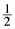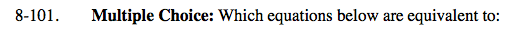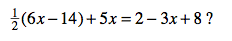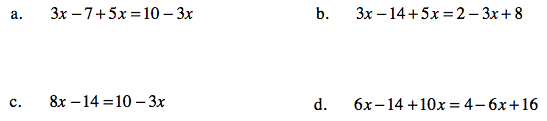### Home > CAAC > Chapter 8 > Lesson 8.3.1 > Problem8-101

8-101.
1. Multiple Choice: Which equations below are equivalent to:(6x −14) + 5x = 2 − 3x + 8? Homework Help ✎

1. 3x − 7 + 5x = 10 − 3x

2. 3x − 14 + 5x = 2 − 3x + 8

3. 8x − 14 = 10 − 3x

4. 6x − 14 + 10x = 4 − 6x + 16Try simplifying each of the equations. Which equations are equivalent?